# Quantile-Quantile Plot Extensions for 'ggplot2'

Extensions of 'ggplot2' Q-Q plot functionalities.

# qqplotrThe `qqplotr` package extends some `ggplot2` functionalities by permitting the drawing of both quantile-quantile (Q-Q) and probability-probability (P-P) points, lines, and confidence bands. The functions of this package also allow a detrend adjustment of the plots, proposed by Thode (2002) to help reduce visual bias when assessing the results.

If you would like to install the development version of `qqplotr`, you may do so by using, for example, `devtools`:

If, instead, you wish to install the stable CRAN version, then simply do:

## Details

The functions of this package, implemeneted as Stats from `ggplot2`, are divided into two groups: (1) Q-Q and (2) P-P plots.

Both groups are composed of three functions: point, line, and band. Those Stats complement each other when drawn together, but they may also be plotted independently.

Below we will give an overview of all those Stats and, further in the document, we will present some usage examples.

### Q-Q plot

• `stat_qq_point` This is a modified version of `ggplot2::stat_qq` with some parameters adjustments and a new option to detrend the points.
• `stat_qq_line` Draws a reference line based on the data quantiles, as in `stats::qqline`.
• `stat_qq_band` Draws confidence bands based on three methods: `"pointwise"`, `"boot"`, `"ks"`, and `"ts"`:
• `"pointwise"` constructs simultaneous confidence bands based on the normal distribution;
• `"boot"` creates pointwise confidence bands based on a parametric boostrap;
• `"ks"` constructs simultaneous confidence bands based on an inversion of the Kolmogorov-Smirnov test;
• `"ts"` constructs tail-sensitive confidence bands, as proposed by Aldor-Noiman et al. (2013).

In order to facilitate the visualization of multiple Q-Q band methods at the same time, the `geom_qq_band` Geom was also implemented. Its usage will be illustrated further below.

### P-P plot

• `stat_pp_point` Plots cumulative probabilities versus probability points. The cumulative probability function is constructed with the sample data, and then evaluated at each probability point.
• `stat_pp_line` Draws a reference identity line ((x = y)).
• `stat_pp_band` Draws confidence bands. For now, only the bootstrap version (`"boot"`) is available.

## Usage

### Q-Q plot

Start by loading the `qqplotr` package:

Let’s start by simulating from a standard Normal distribution:

Then, we use the provided `stat_qq_*` functions to construct a complete Q-Q plot with the points, reference line, and the confidence bands. As default, the standard Q-Q Normal plot with Normal confidence bands is constructed: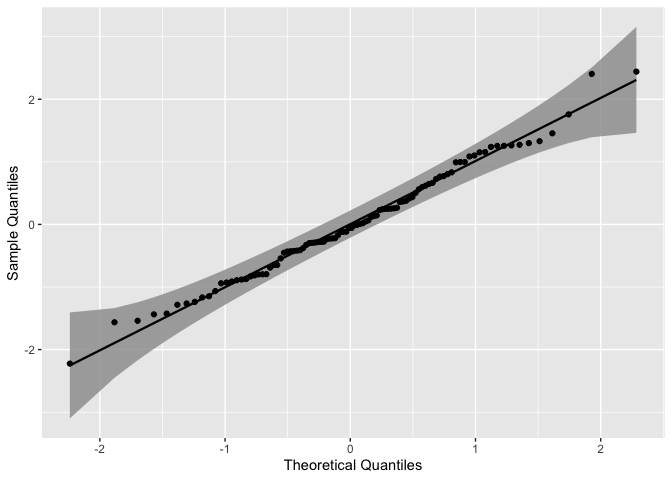As we can see, all the points lie within the confidence bands, which is expected for the given distribution.

As previously described in the Details section, three confidence bands constructs are available, which may be adjusted with the `bandType` parameter. Here, we may use the `geom_qq_band` instead of `stat_qq_band`, which permits a little more flexibility with the graphical parameters when constructing and visualizing different confidence bands.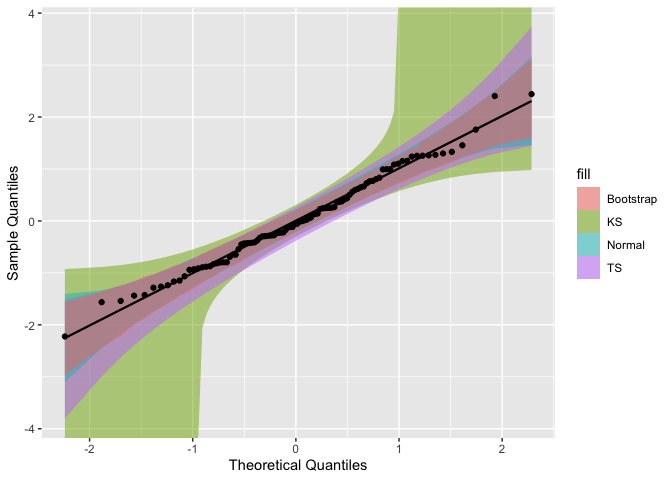To construct Q-Q plots with other theoretical distributions we may use the `distribution` parameter. Specific distributional parameters may be passed as a list to the `dparams` paramater.

Q-Q plots, as changing them will only modify the x-axis range. In contrast, those paramaters will have a higher effect on P-P plots.

Now, let’s use draw the Q-Q plot functions for the mean ozone levels from the `airquality` dataset . Since the data is non-negative, lets choose the Exponential distribution (`exp`) as the theoretical.

It is important to note that the distribution nomenclature follows that from the `stats` package. So, if you wish to provide a custom distribution, you may do so by creating the density, cumulative, quantile, and random functions following the standard nomenclature from the `stats` package, i.e., for the `"custom"` distribution, you must define `"dcustom"`, `"pcustom"`, `"qcustom"`, and `"rcustom"` functions.

That being said, let’s set `distribution = "exp"` and `rate = 2` (the latter one just to exemplify the usage of `dparams`):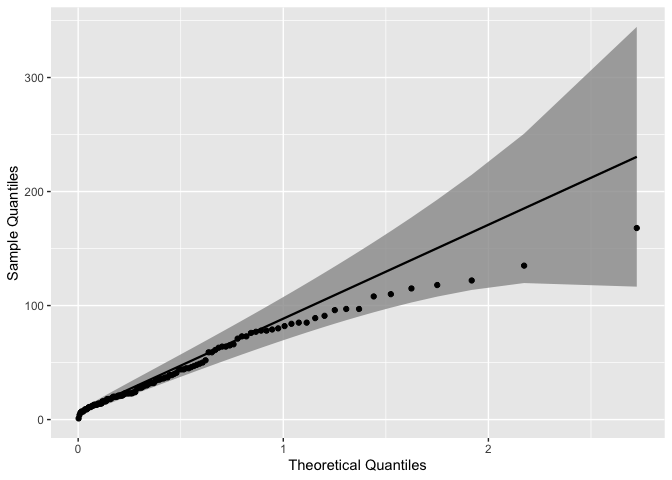The `qqplotr` package also offers the option to detrend Q-Q and P-P plots in order to help reducing visual bias caused by the orthogonal distances from points to the reference lines (Thode, 2002). That bias may cause wrong conclusions to be drawn via visual inference of the plot. To do that we must set `detrend = TRUE`: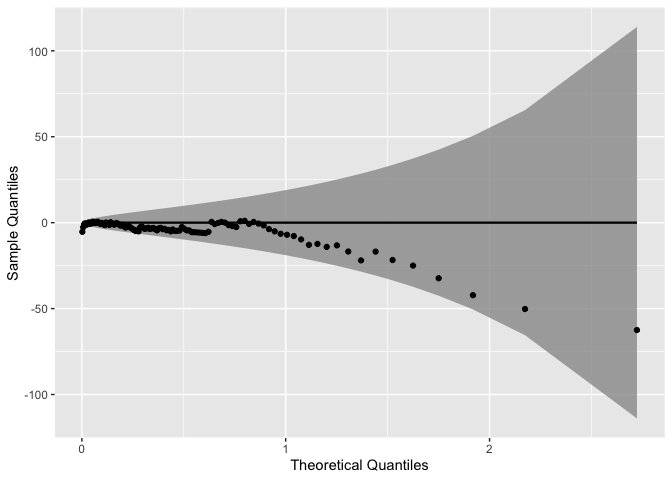Note that the detrend option causes to plot to “rotate”, that is, instead of being presented as a diagonal plot, we visualize the data in a horizontal manner.

The Q-Q plot functions are also compatible with many `ggplot2` operators, such as Facets (sub-plots). For instance, lets consider the barley dataset from the `lattice` package to illustrate how Facets behave when applied to the Q-Q plot functions: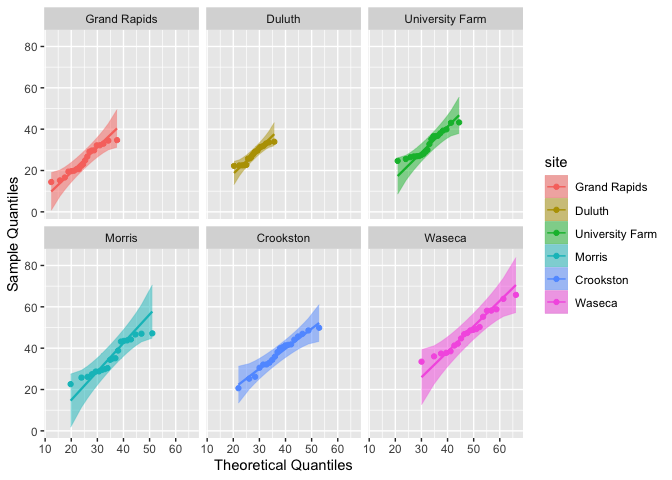### P-P plot

Let’s start by plotting the previously simulated Normal data versus the standard Normal distribution: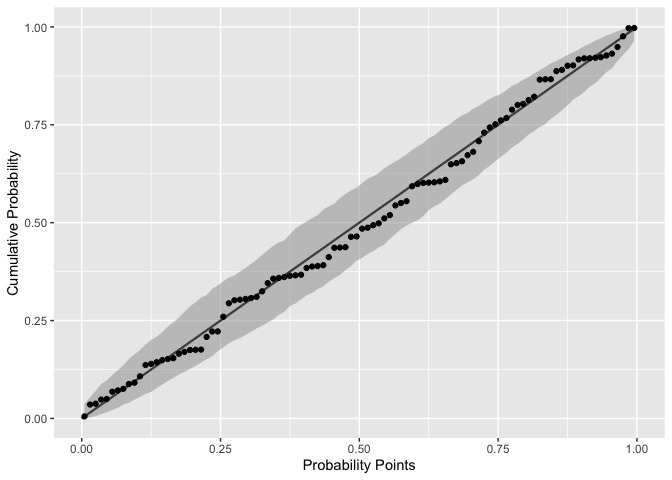Notice that the label names are different from those of the Q-Q plots. Here, the cumulative probability points (y-axis) are constructed by evaluating the theoretical CDF on sample quantiles.

As discussed before, in the case of P-P plots the distributional parameters do impact the results. For instance, say we want to evaluate the same standard Normal data with a shifted and rescaled Normal(2,2) distribution: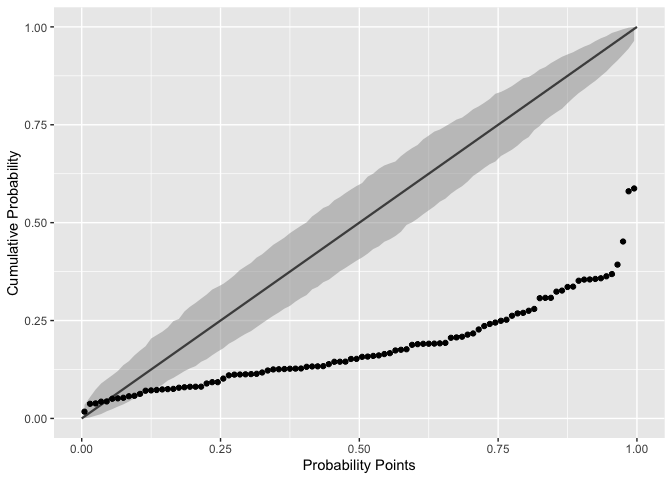As we already know, the plot shows that the chosen Normal distribution parameters are not appropriate for the input data.

Also notice that the `stat_pp_line` function lacks the `dparams` paramater. The reason is that `stat_pp_line` draws by default the identity line and, thus, it isn’t dependent of the sample data and/or its distribution. However, if the user wishes to draw another line (different from the identity) he/she may do so by providing the intercept and slope values, respectively, as a vector of length two to the `ab` parameter: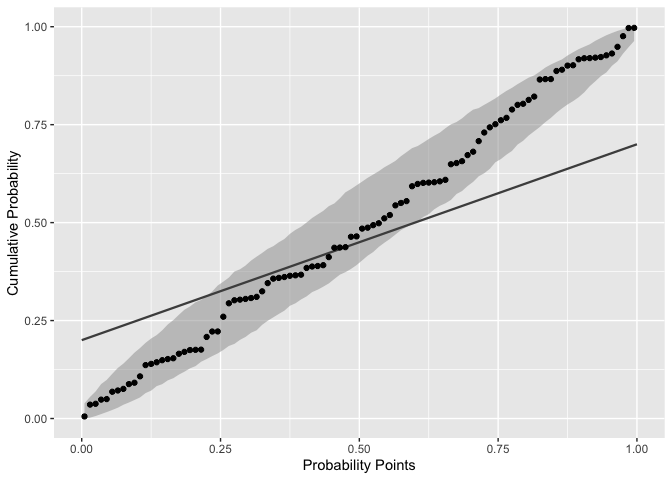We may also detrend the P-P plots in the same way as before. Let’s evaluate again the mean ozone levels from the `airquality` dataset. We had previously seen that the Exponential distribution was more appropriate than the Normal distribution, so let’s take that into account: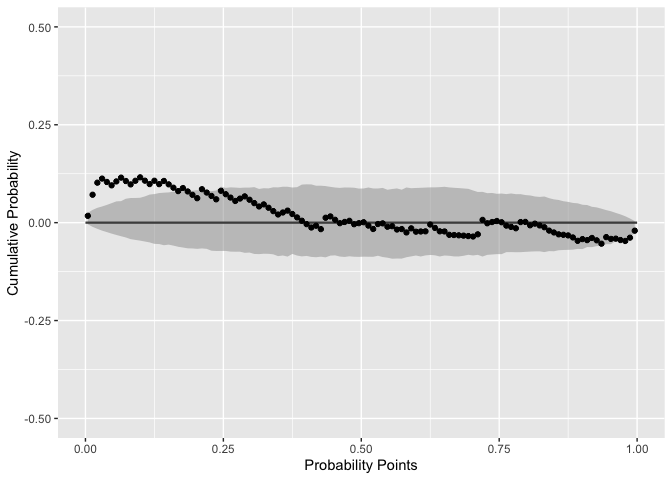Based on empirical tests, we set the rate parameter to `rate = .022`. That value let the most P-P points inside the confidence bands. Even so, that group of outside the confidence bands (at the lower tail) indicate that a more appropriate distribution should be selected.

## Shiny App

In this package, we also included an interactive Shiny app with which you’re able to explore this package functions and its parameters. To run the app, simply call:

# qqplotr 0.0.3

• Added `bandType = "ks"`, which draws simultaneous confidence bands based on an inversion of the Kolmogorov-Smirnov test.

• `bandType = "pointwise"` now produces pointwise confidence bands based on normal theory rather than `bandType = "normal"`.

# qqplotr 0.0.2

• Added the `identity` argument to `*_qq_*` functions, which permits to draw the identity line (instead of the usual Q-Q line).

• Changed `"bs"` to `"boot"` as one of the possible values of the `bandType` argument, for obvious reasons. :)

• By default, distributional parameters are now automatically estimated with MLE for `stat_qq_point`, `stat_qq_line`, `stat_pp_point`, and `stat_pp_band`.

• Created a Shiny app to showcase the package functions and its parameters.

• Included error handling for most of the main parameters from all functions.

• Added partial match for most of the character arguments.

• Fixed a bug caused by `stat_qq_line` default colors when facetting.

• Created the vignette `introduction.Rmd`.

• Removed most inheritances from Q-Q functions, as they were not actually essential.

• Added detrend option for P-P functions.

• Changed the P-P functions labels in the examples from the documentation.

• Implemented the P-P band stat `stat_pp_band` via parametric bootstrap.

• Created a description for the package itself, i.e., now `?qqplotr` works.

• Implemented the P-P plot stats: `stat_pp_point` and `stat_pp_line`.

# qqplotr 0.0.1

• Initial release of the package.

• Implementation of three ggplot2 stats: `stat_qq_point`, `stat_qq_line` and `stat_qq_band`.

# Reference manual

install.packages("qqplotr")

0.0.5 by Adam Loy, 2 months ago

https://github.com/aloy/qqplotr

Report a bug at https://github.com/aloy/qqplotr/issues

Browse source code at https://github.com/cran/qqplotr

Authors: Alexandre Almeida [aut] , Adam Loy [aut, cre] , Heike Hofmann [aut]

Documentation:   PDF Manual

GPL-3 | file LICENSE license

Imports dplyr, robustbase, MASS

Depends on ggplot2

Suggests shiny, devtools, lattice, shinyBS, knitr, rmarkdown

Imported by MKpower, ggResidpanel.

Suggested by HLMdiag, latrend, psycModel, see.

See at CRAN